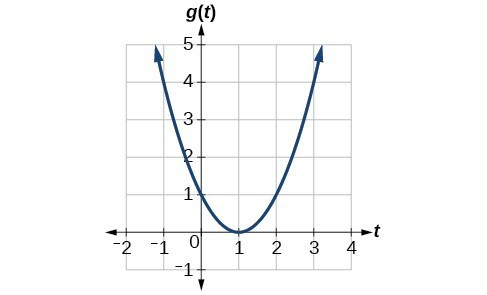## Rates of Change

### Learning Outcomes

• Find the average rate of change of a function.

Gasoline costs have experienced some wild fluctuations over the last several decades. The table below lists the average cost, in dollars, of a gallon of gasoline for the years 2005–2012. The cost of gasoline can be considered as a function of year.

 $y$ 2005 2006 2007 2008 2009 2010 2011 2012 $C\left(y\right)$ 2.31 2.62 2.84 3.3 2.41 2.84 3.58 3.68

If we were interested only in how the gasoline prices changed between 2005 and 2012, we could compute that the cost per gallon had increased from $2.31 to$3.68, an increase of 1.37. While this is interesting, it might be more useful to look at how much the price changed per year. In this section, we will investigate changes such as these. The price change per year is a rate of change because it describes how an output quantity changes relative to the change in the input quantity. We can see that the price of gasoline in the table above did not change by the same amount each year, so the rate of change was not constant. If we use only the beginning and ending data, we would be finding the average rate of change over the specified period of time. To find the average rate of change, we divide the change in the output value by the change in the input value. \begin{align}\text{Average rate of change} &=\frac{\text{Change in output}}{\text{Change in input}}\\[2mm] &=\frac{\Delta y}{\Delta x}\\[2mm] &=\frac{{y}_{2}-{y}_{1}}{{x}_{2}-{x}_{1}}\\[2mm] &=\frac{f\left({x}_{2}\right)-f\left({x}_{1}\right)}{{x}_{2}-{x}_{1}}\end{align} The Greek letter $\Delta$ (delta) signifies the change in a quantity; we read the ratio as “delta-$y$ over delta-$x$” or “the change in $y$ divided by the change in $x$.” Occasionally we write $\Delta f$ instead of $\Delta y$, which still represents the change in the function’s output value resulting from a change to its input value. In our example, the gasoline price increased by1.37 from 2005 to 2012. Over 7 years, the average rate of change was

$\dfrac{\Delta y}{\Delta x}=\dfrac{{1.37}}{\text{7 years}}\approx 0.196\text{ dollars per year}$

On average, the price of gas increased by about 19.6¢ each year.

Other examples of rates of change include:

• A population of rats increasing by 40 rats per week
• A car traveling 68 miles per hour (distance traveled changes by 68 miles each hour as time passes)
• A car driving 27 miles per gallon of gasoline (distance traveled changes by 27 miles for each gallon)
• The current through an electrical circuit increasing by 0.125 amperes for every volt of increased voltage
• The amount of money in a college account decreasing by \$4,000 per quarter

### A General Note: Rate of Change

A rate of change describes how an output quantity changes relative to the change in the input quantity. The units on a rate of change are “output units per input units.”

The average rate of change between two input values is the total change of the function values (output values) divided by the change in the input values.

$\dfrac{\Delta y}{\Delta x}=\dfrac{f\left({x}_{2}\right)-f\left({x}_{1}\right)}{{x}_{2}-{x}_{1}}$

### How To: Given the value of a function at different points, calculate the average rate of change of a function for the interval between two values ${x}_{1}$ and ${x}_{2}$.

1. Calculate the difference ${y}_{2}-{y}_{1}=\Delta y$.
2. Calculate the difference ${x}_{2}-{x}_{1}=\Delta x$.
3. Find the ratio $\dfrac{\Delta y}{\Delta x}$.

### Example: Computing an Average Rate of Change

Using the data in the table below, find the average rate of change of the price of gasoline between 2007 and 2009.

 $y$ 2005 2006 2007 2008 2009 2010 2011 2012 $C\left(y\right)$ 2.31 2.62 2.84 3.3 2.41 2.84 3.58 3.68

The following video provides another example of how to find the average rate of change between two points from a table of values.

### Try It

Using the data in the table below, find the average rate of change between 2005 and 2010.

 $y$ 2005 2006 2007 2008 2009 2010 2011 2012 $C\left(y\right)$ 2.31 2.62 2.84 3.3 2.41 2.84 3.58 3.68

### Example: Computing Average Rate of Change from a Table

After picking up a friend who lives 10 miles away, Anna records her distance from home over time. The values are shown in the table below. Find her average speed over the first 6 hours.

 t (hours) 0 1 2 3 4 5 6 7 D(t) (miles) 10 55 90 153 214 240 282 300

### Example: Computing Average Rate of Change from a Graph

Given the function $g\left(t\right)$, find the average rate of change on the interval $\left[-1,2\right]$.### Example: Computing Average Rate of Change for a Function Expressed as a Formula

Compute the average rate of change of $f\left(x\right)={x}^{2}-\frac{1}{x}$ on the interval $\text{[2,}\text{4].}$

The following video provides another example of finding the average rate of change of a function given a formula and an interval.

### Try It

Find the average rate of change of $f\left(x\right)=x - 2\sqrt{x}$ on the interval $\left[1,9\right]$.

### Example: Finding the Average Rate of Change of a Force

The electrostatic force $F$, measured in newtons, between two charged particles can be related to the distance between the particles $d$, in centimeters, by the formula $F\left(d\right)=\frac{2}{{d}^{2}}$. Find the average rate of change of force if the distance between the particles is increased from 2 cm to 6 cm.

### Example: Finding an Average Rate of Change as an Expression

Find the average rate of change of $g\left(t\right)={t}^{2}+3t+1$ on the interval $\left[0,a\right]$. The answer will be an expression involving $a$.

### Try It

Find the average rate of change of $f\left(x\right)={x}^{2}+2x - 8$ on the interval $\left[5,a\right]$.

## Contribute!

Did you have an idea for improving this content? We’d love your input.